# HSSlive: Plus One & Plus Two Notes & Solutions for Kerala State Board

## AP Board Class 7 Maths Chapter 2 Fractions, Decimals and Rational Numbers Ex 2.3 Textbook Solutions PDF: Download Andhra Pradesh Board STD 7th Maths Chapter 2 Fractions, Decimals and Rational Numbers Ex 2.3 Book AnswersAP Board Class 7 Maths Chapter 2 Fractions, Decimals and Rational Numbers Ex 2.3 Textbook Solutions PDF: Download Andhra Pradesh Board STD 7th Maths Chapter 2 Fractions, Decimals and Rational Numbers Ex 2.3 Book Answers

## Andhra Pradesh State Board Class 7th Maths Chapter 2 Fractions, Decimals and Rational Numbers Ex 2.3 Books Solutions

 Board AP Board Materials Textbook Solutions/Guide Format DOC/PDF Class 7th Subject Maths Chapters Maths Chapter 2 Fractions, Decimals and Rational Numbers Ex 2.3 Provider Hsslive

## How to download Andhra Pradesh Board Class 7th Maths Chapter 2 Fractions, Decimals and Rational Numbers Ex 2.3 Textbook Solutions Answers PDF Online?

2. Click on the Andhra Pradesh Board Class 7th Maths Chapter 2 Fractions, Decimals and Rational Numbers Ex 2.3 Answers.
3. Look for your Andhra Pradesh Board STD 7th Maths Chapter 2 Fractions, Decimals and Rational Numbers Ex 2.3 Textbooks PDF.
4. Now download or read the Andhra Pradesh Board Class 7th Maths Chapter 2 Fractions, Decimals and Rational Numbers Ex 2.3 Textbook Solutions for PDF Free.

## AP Board Class 7th Maths Chapter 2 Fractions, Decimals and Rational Numbers Ex 2.3 Textbooks Solutions with Answer PDF Download

Find below the list of all AP Board Class 7th Maths Chapter 2 Fractions, Decimals and Rational Numbers Ex 2.3 Textbook Solutions for PDF’s for you to download and prepare for the upcoming exams:

Question 1.
Fill ¡n the blanks in the table. One is done for you.

 Division Quotient 1. 362.21 ÷ 10 36.221 2. 5636.1 ÷ 100 56.361 3. 374.9 ÷ 1000 0.3749 4. 2016.4 ÷ 1000 2.0164 5. 123.0 ÷100 1.23 6. 1300.7 ÷ 1000 1.3007 7. 590.01 ÷ 10 59.001

Question 2.
Solve the following,
(i) 5.51 ÷ 2
5.51 ÷ 2
551100÷21
551100×12
551×1100×2
551200
∴ 5.51 ÷ 2 = 2.755

(ii) 38.4 ÷ 3
38.4 ÷ 3
38410÷31
38410×13
384×110×3
38430
∴ 38.4 ÷ 3 = 12.8

(iii) 57.39 ÷ 6
57.39 ÷ 6
5739100÷61
5739100×16
5739×1100×6
5739600
∴ 57.39 ÷ 6 = 9.565

(iv) 562.1 ÷ 11
562.1 ÷ 11
562110÷111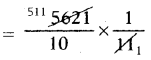51110
∴ 562.1 ÷ 11 = 51.1

(v) 0.7005 ÷ 5
0.7005 ÷ 5
700510000÷51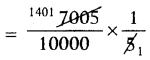140110000
∴ 0.7005 ÷ 5 = 0.1401

(vi) 9.99 ÷ 3
9.99 ÷ 3
999100÷31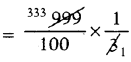333100
∴ 9.99 ÷ 3 = 3.33

(vii) 13 ÷ 6.5
13 ÷ 6.5
131÷6510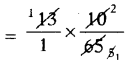21
∴ 13 ÷ 6.5 = 2

(viii) 10.01 ÷ 11
10.01 ÷ 11
1001100÷111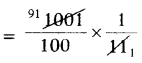91100
∴ 10.01 ÷ 11 = 0.91

(ix) 8 ÷ 0.32
8 ÷ 0.32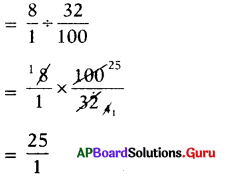∴ 8 ÷ 0.32 = 25

(x) 320.1 ÷ 33
320.1 ÷ 33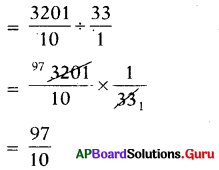∴ 320.1 ÷ 33 = 9.7

Question 3.
Solve the following divisions,
(i) 78.24 ÷ 0.2
78.24 ÷ 0.2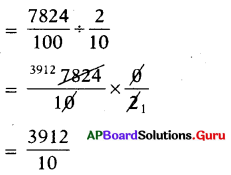∴ 78.24 ÷ 0.2 = 391.2

(ii) 4.845 ÷ 1.5
4.845 ÷ 1.5
48451000÷1510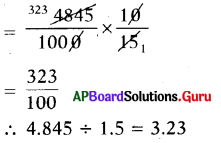(iii) 0.246 ÷ 0.6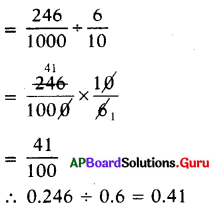(iv) 563.2 ÷ 2.2
563.2 ÷ 2.2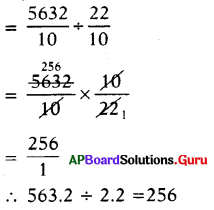∴ 563.2 ÷ 2.2 = 256

(v) 0.026 ÷ 0.13
0.026 ÷ 0.13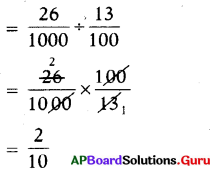∴ 0.026 ÷ 0.13 = 0.2

(vi) 4.347 ÷ 0.09
4.347 ÷ 0.09
43471000÷9100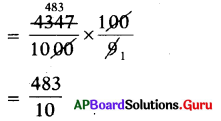∴ 4.347 ÷ 0.09 = 48.3

(vii) 3.9 ÷ 0.13
3.9 ÷ 0.13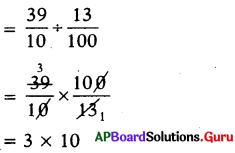∴ 3.9 ÷ 0.13 = 30

(viii) 2032 ÷ 0.8
20.32 ÷ 0.8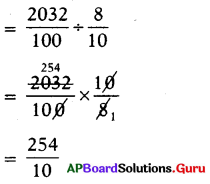∴ 20.32 ÷ 0.8 = 25.4

(ix) 24.4 ÷ 6.1
24.4 ÷ 6.1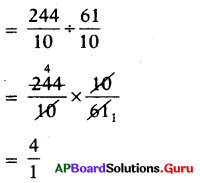∴ 24.4 ÷ 6.1 = 4

(x) 2.164 ÷ 0.008
2.164 ÷ 0.008
21641000÷81000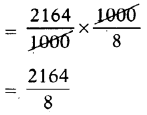∴ 2.164 ÷ 0.008 = 270.5

Question 4.
Solve the following.
(i) Divide 39.54 by 6
39.54 by 6
= 39.54 ÷ 6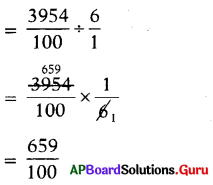∴ 39.54 ÷ 6 = 6.59

(ii) Divide 72 by 10
7.2 by 10
= 7.2 ÷ 10
7210÷101
7210×110
72×110×10=72100
∴ 7.2 ÷ 10 = 0.72

(iii) Divide 5.2 by 1.3
5.2 by 1.3
= 5.2 ÷ 1.3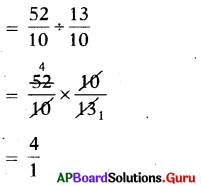∴ 5.2 ÷ 1.3 = 4

Question 5.
‘Sekhar travelled 154.5 km. in 5 hours with uniform speed on his bike. How much distance does he travel in one hour ?
Given, distance travelled in 5 hours = 154.5 km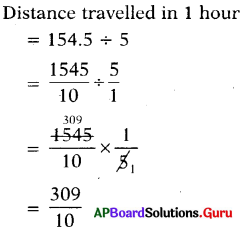∴ Distance travelled by Sekhar in 1 hour = 30.9 km

Question 6.
If a Mason worked 100 hours in 12.5 days to construct a wall, then how many hours he totally worked in a day?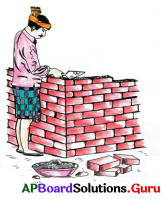Given number of hours worked in 12.5 days to.construct a wall = 100 hours.
Number of hours worked in one day
= 100 ÷ 12.5
1001÷12510
1001×10125
1000125
∴ Number of hours worked by Mason in one day = 8 hours.

Question 7.
If the cost of dozen eggs is ₹ 61.80, then find the cost of an egg.
Given, cost of dozen eggs = ₹ 61.80
We know 1 dozen =12
Cost of one egg = 61.80 ÷ 12515100
∴ Cost of one egg = ₹ 5.15

Question 8.
If the price of a tablet strip containing 10 tablets is ₹ 26.5, then find the price of each tablet.
Given, cost of 10 tablets = ₹ 26.5
Cost of each tablet = 26.5 ÷ 10
26510÷101
26510×110
265100

∴ Cost of each tablet = ₹ 2.65

## Andhra Pradesh Board Class 7th Maths Chapter 2 Fractions, Decimals and Rational Numbers Ex 2.3 Textbooks for Exam Preparations

Andhra Pradesh Board Class 7th Maths Chapter 2 Fractions, Decimals and Rational Numbers Ex 2.3 Textbook Solutions can be of great help in your Andhra Pradesh Board Class 7th Maths Chapter 2 Fractions, Decimals and Rational Numbers Ex 2.3 exam preparation. The AP Board STD 7th Maths Chapter 2 Fractions, Decimals and Rational Numbers Ex 2.3 Textbooks study material, used with the English medium textbooks, can help you complete the entire Class 7th Maths Chapter 2 Fractions, Decimals and Rational Numbers Ex 2.3 Books State Board syllabus with maximum efficiency.

## FAQs Regarding Andhra Pradesh Board Class 7th Maths Chapter 2 Fractions, Decimals and Rational Numbers Ex 2.3 Textbook Solutions

#### Can we get a Andhra Pradesh State Board Book PDF for all Classes?

Yes you can get Andhra Pradesh Board Text Book PDF for all classes using the links provided in the above article.

## Important Terms

Andhra Pradesh Board Class 7th Maths Chapter 2 Fractions, Decimals and Rational Numbers Ex 2.3, AP Board Class 7th Maths Chapter 2 Fractions, Decimals and Rational Numbers Ex 2.3 Textbooks, Andhra Pradesh State Board Class 7th Maths Chapter 2 Fractions, Decimals and Rational Numbers Ex 2.3, Andhra Pradesh State Board Class 7th Maths Chapter 2 Fractions, Decimals and Rational Numbers Ex 2.3 Textbook solutions, AP Board Class 7th Maths Chapter 2 Fractions, Decimals and Rational Numbers Ex 2.3 Textbooks Solutions, Andhra Pradesh Board STD 7th Maths Chapter 2 Fractions, Decimals and Rational Numbers Ex 2.3, AP Board STD 7th Maths Chapter 2 Fractions, Decimals and Rational Numbers Ex 2.3 Textbooks, Andhra Pradesh State Board STD 7th Maths Chapter 2 Fractions, Decimals and Rational Numbers Ex 2.3, Andhra Pradesh State Board STD 7th Maths Chapter 2 Fractions, Decimals and Rational Numbers Ex 2.3 Textbook solutions, AP Board STD 7th Maths Chapter 2 Fractions, Decimals and Rational Numbers Ex 2.3 Textbooks Solutions,
Share: